### 2.2.3.1 General principles of micromagnetics

The process of demagnetization in a standard hysteresis experiment consists basically of a series of stationary states. The time of measurement (typically miliseconds) is as large as to disregard rapid dynamic effects (occurring at pico - nanoseconds). For this kind of process the micromagnetic approach, firstly introduced by W.F. Brown [Brown 63a], is used. The micromagnetism is a semiclassical approach that considers the magnetizationas a vector function of the spatial coordinates, but with constant module. This implies a continuous approximation that ignores any discrete effect. The magnitudeis the saturation magnetization and it is defined as the density of magnetic moments (per unit of volume in the material). It is more useful to work with the magnetization unit vector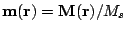. The energies appearing in the atomistic level are substituted by a functional of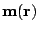:(2.8)(2.9)(2.10)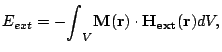(2.11)(2.12)

The micromagnetic exchange energy is a continuous version of the atomistic exchange. The expression is the square of a derivative and, therefore, the minimum value corresponds to the saturated stated. Therefore, the micromagnetic exchange also favors all the moments to be parallel. For granular magnetic material the exchange constant is often phenomenological.

The atomistic magnetic moment and the saturation magnetization are related through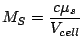(2.13)

whereis the volume of the unit cell and c the number of moments per cell(for sc,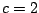for bcc,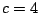for fcc). The anisotropy constant is an energy density, whose origin is equivalent to the atomistic anisotropy and their relation is: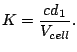(2.14)

The magnetization state is found by minimizing the total energy with respect to the function. Namely, we must solvewith the restriction. The minimum found by this procedure is normally a relative minimum of the total energy. The minimization procedure depends on the initial conditions and the final state is dependent of the initial state. On the other hand, this is also the case in the experiments. The variational problem solution satisfies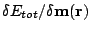=0 with the addition of a Lagrange multiplier term, proportional to. Therefore, the solution of variational problem also satisfies the Brown condition for minimum: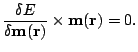We can define an effective field as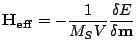(2.15)

and to rewrite the Brown condition as(2.16)

From Eq. (2.8) the total effective field is a sum of exchange, anisotropy, external and magnetostatic fields: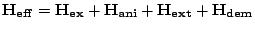(2.17)

with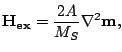(2.18)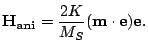(2.19)

It is often useful to define the anisotropy field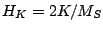and normalize all the fields to this parameter.

Until the rapid development of the computers, the micromagnetics was an analytical theory and many analytical results were derived. However, normally all of them are based on simplifications and have very limited regions of applicability. In numerical approaches we divide or discretize the sample by the discretization length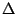, considering a constant magnetization inside each element.

There are two physically relevant parameters that we need to take in account in order to select the size of the discretization length: the wall-width parameter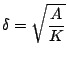(2.20)

and the exchange length(2.21)

The domain wall width parameter determines the thickness of the domain wall separating magnetic domains of different magnetization directions. This parameter corresponds to the width of a Bloch wall that is found in materials in which the main source of anisotropy is magnetocrystalline anisotropy (hard materials). The exchange length is the length below which atomic exchange interactions dominate typical magnetostatic fields. The exchange length is proportional to the Néel domain wall width. This type of domain wall that only contains volume charges appears in thin films of soft magnetic material. In order to describe correctly the domain wall the discretization length must be less than its width but include enough atoms to be valid as the continuous approximation.

In the literature there are two main methods of discretization: Finite Differences (FD) [Berkov 93] and Finite Elements Method (FEM) [Fidler 00]. The advantage of the finite differences method is its easy implementation but its main disadvantage is its inability to describe irregular shapes. To overcome this problem Gibbons proposed the Embedded Curved Boundary (ECB) method as an alternative based on the interpolation of the boundary elements [Gibbons 00]. For curved surfaces FEM is best suited because its elements are tetrahedra with variable size , although any numerical approach will fail to describe real surfaces. At the present, there are several software packages available for micromagnetic simulations. Most of them are commercial programs, but also there have been efforts to release free source codes in particular Oommf [OOMMF ], which uses FD method with Fast Fourier Transform, and magpar [Magpar ], which uses FEM simulations. In our calculations in the following chapters we used codes developed inside the group that use the finite differences method.

In the numerical solution of Eq. (2.16) the right term can never be zero due to numerical convergence problem. We want this value to be as low as possible, but in practice we finish our calculation when a tolerance value has been reached. In our calculation we use the following definition for the micromagnetic error: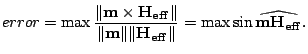(2.22)

We will consider that calculations are properly converged when. A reasonable value ofis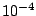.

Finally, we will return to the micromagnetic exchange constant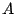. Its relation with the atomistic exchange parameter can be easy calculated if we compare the finite difference version of Eq. (2.18) and the effective field arising from Eq. (2.5) and makingequal to the lattice distance. Thus in a tetragonal with lattice size parameters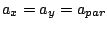and:(2.23)(2.24)

In the case of a cubic cell the equivalence becomes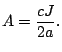(2.25)

2008-04-04